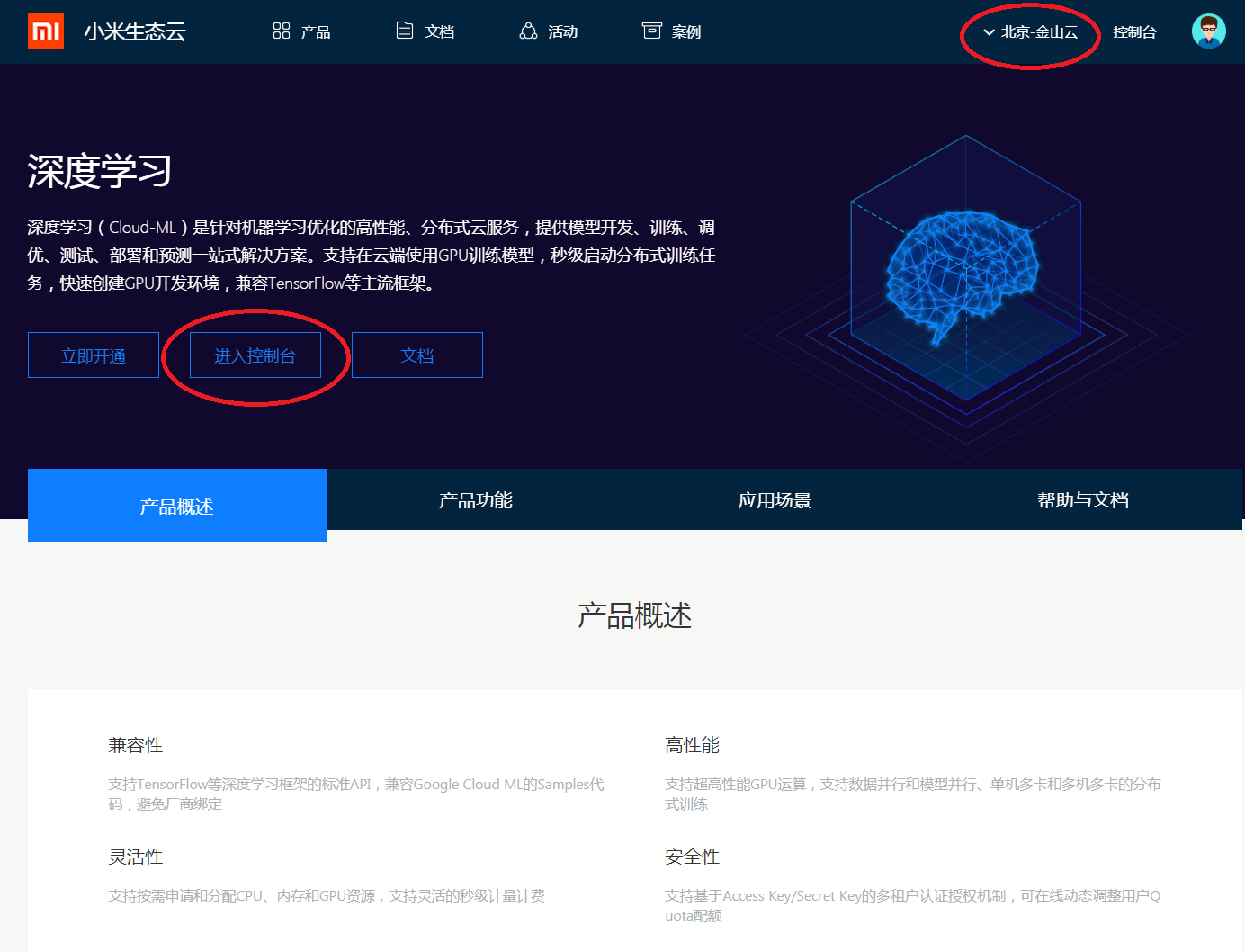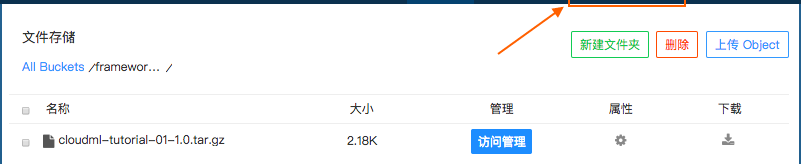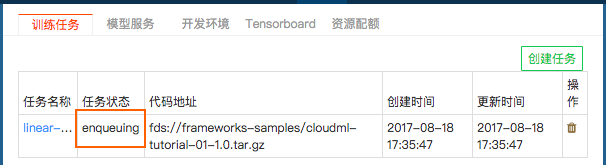## 上手 Trainjob

1. 怎样用命令行和 Web 提交训练任务；
2. 怎样查看事件和 Log；
3. 怎样查看提交的训练任务；
4. 怎样删除任务；

### 示例介绍### 代码准备

``````.
|____simple_tf_regress
| |____setup.py          # （可选，用于项目代码的构建和分发）
| |____requirements.txt  # （可选，用于记录项目可能包含的 Python 依赖）
| |____trainer
| | |______init__.py
``````

• `setup.py` 内容如下：

``````  import setuptools
setuptools.setup(name='cloudml-tutorial-01', version='1.0', packages=['trainer'])
``````

其中，`name`是压缩包的名字，`version`是训练代码的版本，`packages`指定训练代码所依赖的其他第三方函数库。如果指定，Cloud-ML平台会在创建的容器中先进行安装。

关于如何编写 `setup.py`, 详情请参考 Python 文档

• `requirements.txt` 保存可用 `pip` 安装的依赖，如果指定，Cloud-ML 平台会在执行训练之前先进行安装。

更多详情请参考 Pip 文档

• `trainer` 为训练的 Python package；
• `__init__.py` Python package 文件，详情请参考 Python 文档
• `task.py`内容为上面的训练代码；

``````python -m trainer.task
``````

### 使用命令行提交任务

``````➜  ~ cloudml -v
0.2.11
``````

``````cloudml jobs submit -n linear -m trainer.task -u ./simple_tf_regress -d cnbj6-repo.cloud.mi.com/cloud-ml/tensorflow-gpu:1.7.0-xm1.0.0 -c 0.5 -M 100M
``````

Required Argument Type Example
Yes -n --name string linear
Yes -u --trainer_uri string ./simple_tf_regress
No -c --cpu_limit int 0.5
No -M --memory_limit string 100M
No -d --docker_image string cnbj6-repo.cloud.mi.com/cloud-ml/tensorflow-gpu:1.7.0-xm1.0.0

• `-cMd`三个参数是可选参数，如果用户不指定，命令行程序会自动用默认填充，但在实际提交给平台的时候仍然是必须的参数;
• `-u --trainer_uri` 支持训练项目的本地相对路径或绝对路径，同时也支持用户打包上传到 FDS 后的文件链接（主要用于 Web 提交任务）；

注1： 客户端支持 `-u` 指定本地项目路径，实现逻辑是客户端自动打包上传到 FDS `cloudml_default_fds_bucket` 下的 `user_packages/` 路径里，上传包名会添加时间信息后缀，使用路径如不存在会尝试创建，因此，为了该功能正常工作，需要用户对配置的 `cloudml_default_fds_bucket` 可写， 更多关于 `cloudml_default_fds_bucket` 的说明，参考：使用fuse

注2： 后续章节中可能选取本地路径或 FDS 链接两种支持方式中的其中一种举例，如无特殊情况，不再做重复说明，用户可自行选取适合方式；

• 更多详细的参数帮助信息，请使用 `cloudml jobs submit -h` 查看。

``````cloudml jobs list
``````

``````cloudml jobs logs linear
``````

`cloudml jobs logs` 高级选项：• `-f, --follow` 提供对训练容器日志的跟随查看模式，类似 `tail` 命令的 `-f`，实时展示最新日志流；
• `-n LINES, --lines LINES` 支持整数参数，用来控制返回日志中的最后 `LINES` 行，不指定默认返回从训练容器被创建开始的所有日志，通过 `-n` 方便用户在大量输出情况下控制输出的内容数量；
• `-f``-n LINES` 可以组合使用；

1. Log的产生在容器成功创建之后，所以有时候查看Log的时候，会看到提示“容器还未创建”，这时候需要等待一小会儿，如果时间过长（比如>5分钟）则很可能容器创建出错，请联系管理员。
2. 在任务结束以后，系统会对容器的Log保存一段时间，超过这个时间的容器的Log会被删除。

Cloud-ML平台底层依赖Kubernetes对容器进行管理。容器的生命周期的变迁涉及不同的状态，状态的变迁对应不同事件。Cloud-ML提供了接口可以方便用户查看容器的事件。 一般来说，用户并不太关心更底层的操作，除非如上面介绍，当Log查看出问题的时候，可以通过平台提供的事件接口查看更底层的操作。

``````cloudml jobs events linear
````````````cloudml jobs delete linear
``````

### 使用Web界面提交任务``````python setup.py sdist --format=gztar
``````

``````.
|____simple_tf_regress
| |____cloudml_tutorial_01.egg-info
| | |____PKG-INFO
| | |____SOURCES.txt
| | |____top_level.txt
| |____dist
| | |____cloudml-tutorial-01-1.0.tar.gz
| |____setup.py
| |____trainer
| | |______init__.py
| | |______init__.pyc
``````

``````cd ..
tar -czf simple_tf_regress.tar.gz simple_tf_regress
``````

FDS 可以使用命令行或 Web 界面上传，详情请参考FDS文档。 这里假定已经上传到下面目录：### 附录1，示例代码

``````'''
A linear regression learning algorithm example using TensorFlow library.
Author: Aymeric Damien
Project: https://github.com/aymericdamien/TensorFlow-Examples/
'''

from __future__ import print_function

import tensorflow as tf
import numpy
rng = numpy.random

# Parameters
learning_rate = 0.01
training_epochs = 1000
display_step = 50

# Training Data
train_X = numpy.asarray([3.3,4.4,5.5,6.71,6.93,4.168,9.779,6.182,7.59,2.167,
7.042,10.791,5.313,7.997,5.654,9.27,3.1])
train_Y = numpy.asarray([1.7,2.76,2.09,3.19,1.694,1.573,3.366,2.596,2.53,1.221,
2.827,3.465,1.65,2.904,2.42,2.94,1.3])
n_samples = train_X.shape

# tf Graph Input
X = tf.placeholder("float")
Y = tf.placeholder("float")

# Set model weights
W = tf.Variable(rng.randn(), name="weight")
b = tf.Variable(rng.randn(), name="bias")

# Construct a linear model

# Mean squared error
cost = tf.reduce_sum(tf.pow(pred-Y, 2))/(2*n_samples)
#  Note, minimize() knows to modify W and b because Variable objects are trainable=True by default

# Initializing the variables
init = tf.global_variables_initializer()

# Launch the graph
with tf.Session() as sess:
sess.run(init)

# Fit all training data
for epoch in range(training_epochs):
for (x, y) in zip(train_X, train_Y):
sess.run(optimizer, feed_dict={X: x, Y: y})

# Display logs per epoch step
if (epoch+1) % display_step == 0:
c = sess.run(cost, feed_dict={X: train_X, Y:train_Y})
print("Epoch:", '%04d' % (epoch+1), "cost=", "{:.9f}".format(c), \
"W=", sess.run(W), "b=", sess.run(b))

print("Optimization Finished!")
training_cost = sess.run(cost, feed_dict={X: train_X, Y: train_Y})
print("Training cost=", training_cost, "W=", sess.run(W), "b=", sess.run(b), '\n')

# Testing example, as requested (Issue #2)
test_X = numpy.asarray([6.83, 4.668, 8.9, 7.91, 5.7, 8.7, 3.1, 2.1])
test_Y = numpy.asarray([1.84, 2.273, 3.2, 2.831, 2.92, 3.24, 1.35, 1.03])

print("Testing... (Mean square loss Comparison)")
testing_cost = sess.run(
tf.reduce_sum(tf.pow(pred - Y, 2)) / (2 * test_X.shape),
feed_dict={X: test_X, Y: test_Y})  # same function as cost above
print("Testing cost=", testing_cost)
print("Absolute mean square loss difference:", abs(
training_cost - testing_cost))
``````

### 附录2，Trainjob状态图

Cloud-Ml Trainjob有下面几种状态：### 附录3，获取"密钥ID"